## Car A is traveling north on a straight highway and car B is traveling west on a different straight highway. Each car is approaching the inte

Question

Car A is traveling north on a straight highway and car B is traveling west on a different straight highway. Each car is approaching the intersection of these highways. At a certain moment, car A is 0.3 km from the intersection and traveling at 95 km/h while car B is 0.4 km from the intersection and traveling at 90 km/h. How fast is the distance between the cars changing at that moment? km/h

in progress 0
6 months 2021-08-09T11:21:40+00:00 2 Answers 15 views 0

129 km/hr

Explanation:

Distance of Car A North of the Intersection, y=0.3km

Distance of Car B West of the Intersection, x=0.4 km

The distance z,  between A and B is determined by the Pythagoras theorem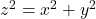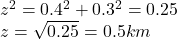Taking derivative of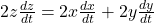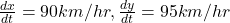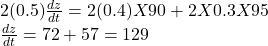The distance z, between the cars is changing at a rate of 129 km/hr.

The rate at which the distance changes at that moment is 91.743  Km/h

Explanation:

The time for car a to get to the intersection =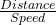=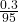= 0.00316 h

The time for car B to get to the intersection ==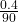= 0.00444 h

Total time =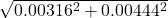= 0.00545 h

Total distance between the two cars at that moment =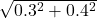= 0.5 Km

The rate at which the distance between the cars is changing =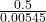=  91.743  Km/h